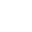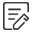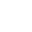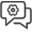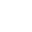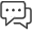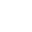RECOMMEND NEWS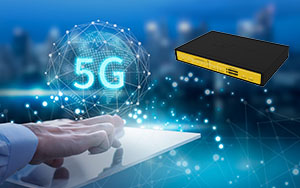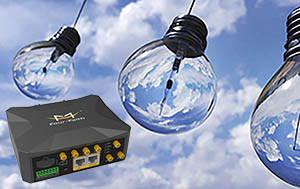FEATURED PRODUCT

Date:2022-08-03 16:37:18

### How Good is the Signal Strength of RSRP? Introduction of RSRQ Signal Strength

RSRQ (Reference Signal Receiving Quality) represents the receiving quality of the LTE reference signal. This metric is mainly used to sort different LTE candidate cells according to the signal quality. Such measurements are used as input for handover and cell reselection decisions.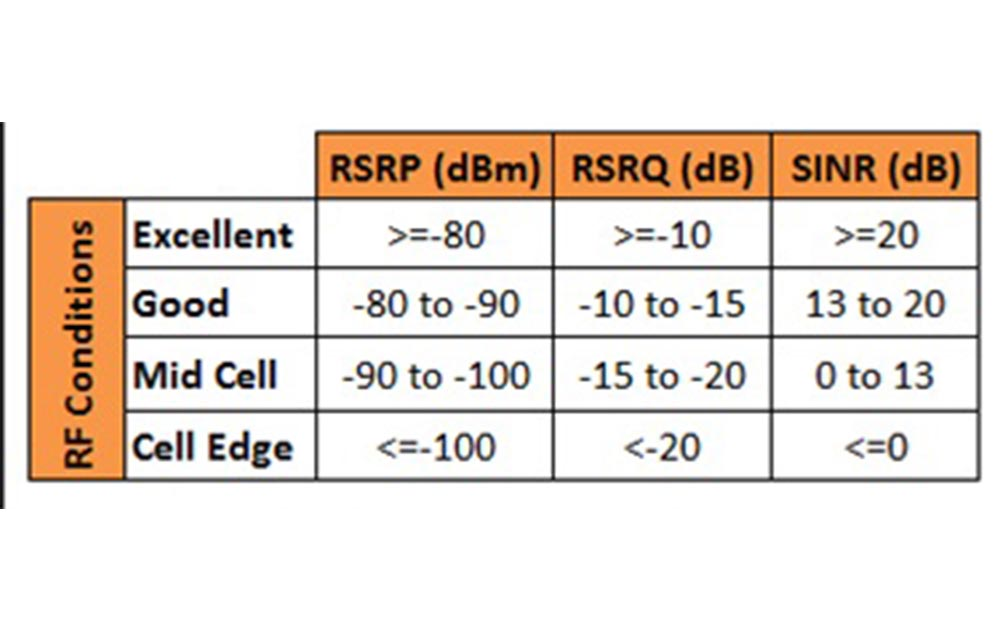1. Introduction of RSRQ signal strength

RSRQ signal strength is defined as the ratio of N*RSRP/(LTE carrier RSSI), where N is the number of resource blocks (RBs) of the LTE carrier RSSI measurement bandwidth. RSRQ signal strength achieves an efficient way to report the combined effect of signal strength and interference.

2. RSRQ signal strength range: -19.5dB(bad) to -3dB (good).

3. RSRP signal strength

RSRP signal strength is better than negative 85dBm signal. rsrp is one of the key parameters and physical layer measurement requirements that can represent wireless signal strength in LTE networks. It is the average value of the signal power received on all REs carrying the reference signal within a certain symbol. Both usefulness and specification are equivalent to RSCP received signal code power in WCDMA.

A signal is a physical quantity that represents a message. For example, an electrical signal can represent different messages through changes in amplitude, frequency, and phase. There are two types of electrical signals: analog signals and digital signals. A signal is a tool for carrying messages and a carrier of messages. Broadly speaking, it includes optical signals, acoustic signals and electrical signals, etc. According to the actual use, the signals include TV signals, broadcast signals, radar signals, communication signals, etc.; according to the time characteristics, there are deterministic signals and random signals.

A signal is a tool for carrying messages and a carrier of messages. Broadly speaking, it includes optical signals, acoustic signals and electrical signals, etc. For example, the ancients used the billowing smoke produced by lighting the beacon tower to transmit the news of the enemy's invasion to the distant army, which belongs to the light signal; when we speak, the sound wave is transmitted to the ears of others, so that others understand our intention, which belongs to the sound. Signal.

4. Introduction of RSRP, RSSI, SINR

4.1 RSRP (Reference Singnal Received Power, Reference Signal Received Power) is the cell common reference signal (CRS) power value received by the terminal. The value is the linear average of the power of a single RE within the measurement bandwidth, which reflects the usefulness of this cell. the strength of the signal.

4.2 RSSI (Received Singnal Strengthen Indicator, Received Signal Strength Indicator) is the linear average value of the power of all signals received by the terminal (including useful and interference on the same frequency, adjacent frequency interference, thermal noise, etc.) The load intensity on the resource.

4.3 SINR (Signal to Interference & Noise Ratio) is the ratio of useful signal power to the sum of interference and noise power, which directly reflects the quality of the received signal.